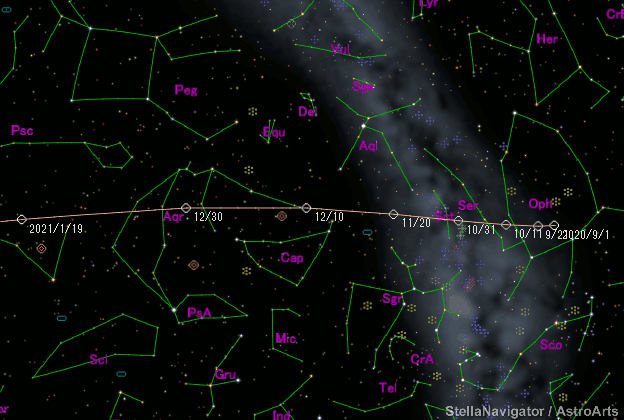# \$B%^%C%/%[%k%DBh#2WB@1(B

141P/Machholz 2 (2020)###\$B###\$B50F;MWAG(B

```                    Epoch = 2020 Dec. 17.0 TT
T = 2020 Dec. 16.26997 TT        Peri. = 153.54399
e = 0.7356668                    Node  = 241.84951 2000.0
q = 0.8079340 AU                 Incl. =  13.94456
a =  3.0564980 AU   n = 0.18444543   P =   5.34 years
```

###\$B@1?^(B###\$B8wEYJQ2=(B

```        m1 = 13.5 + 5 log\$B&\$(B + 25 log r(t - 15)
```##### \$B50F;MWAG\$O(BCBET 4834\$B\$K7G:\\$5\$l\$?\$b\$N\$G\$9!#(B \$B@1?^\$O%9%F%i%J%S%2!<%?(B Ver.10 (\$B%"%9%H%m%"!<%D(B) \$B\$G:n@.\$7\$?\$b\$N\$G\$9!#(B \$B8wEY%0%i%U\$O(BComet for Windows\$B\$G:n@.\$7\$?\$b\$N\$G\$9!#(B